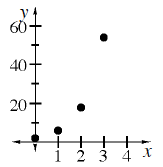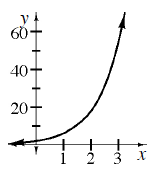### Home > CCA2 > Chapter B > Lesson B.1.6 > ProblemB-74

B-74.

A particular sequence can be represented by $t(n)=2(3)^n$.

1. What are $t(0)$, $t(1)$,$t(2)$, and $t(3)$?

$2, 6, 18, 54$

2. Graph this sequence. What is the domain?Domain: non-negative integers

3. On the same set of axes, graph the function $f(x)=2(3)^x$ .4. How are the two graphs similar? How are they different?

How do their shapes compare?
Are they discrete or continuous?